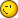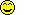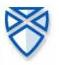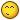# resolution equation x^2

7 replies to this topic

### #1abelmartin

abelmartin

Newbie

•• Members
•• 23 posts
• Location:Spain
• Interests:Calculators collection

• Calculators:

Posted 27 June 2004 - 11:09 AM

?C?mo se puede resolver, con la opci?n de ?lgebra simb?lica, el sistema formado por las ecuaciones:
x-y=3
x^2+y=8
yo lo estoy resolviendo por m?todos gr?ficos pero me gustar?a conocer alg?n m?todo algebraico.
Un saludoHow it is possible to be solved, with the option of symbolic algebra, the system formed by the equations: x-y=3 x^2+y=8 I am solving it by graphical methods but I would like to know some method algebraic. A greeting

Edited by abelmartin, 27 June 2004 - 03:26 PM.

### #2jlssoft

jlssoft

Newbie

•• Validating
•• 6 posts
• Location:Mor?n de la Frontera-Sevilla. Spain

• Calculators:

Posted 27 June 2004 - 12:53 PM

Saludos Abel:
Primero te dir? que el sistema que propones lo soluciona sin problemas la TI voyage 200 con la orden solve(x^2+y=8 and x-y=3,x) como se ve la sintaxis cambia un poco. Con esta orden te da las dos soluciones del sistema. El hecho de que la Classpad no sea capaz de resolverlo creo que se puede deber a que todav?a hay que pulir su software. A m? lo ?nico que se me ocurre de momento es utilizar:
solve(x^2+y=8,y)|x=y+3 con lo que sale una ecuaci?n con y como inc?gnita que se puede resolver con la misma orden solve.
De todas formas en ?sto t? eres el maestro, as? que si encuentras algo por ah? pues nos lo dices.
Suerte en el ICME.### #3Andy.Davies

Andy.Davies

Forum Ghost

•• [Legends]
•• 1547 posts
• Gender:Male
• Location:Dorset, England
• Interests:Age: 18
Studying: MEng in cybernetics at Reading uni
Interests: Progaming (VB, VB.Net, C#, Casio Basic)
Computers UBBD (Using, Building, Breaking &amp; Destroying)
Gaming (FPS, RTS, RPG)
Electronics
Rock Music (Preferably Loud)
Riley's (Pool &amp; Snooker Bar)
Driving (Preferably fast)
Aikedo (Martial Art)

• Calculators:
Algebra FX 2.0 ROM 1.01, FX9750G

Posted 27 June 2004 - 01:07 PM

Please remember to put a translation of your post at the bottom of the post. Thanks### #4abelmartin

abelmartin

Newbie

•• Members
•• 23 posts
• Location:Spain
• Interests:Calculators collection

• Calculators:

Posted 27 June 2004 - 03:05 PM

Hago lo que me comentas y no me sale nada, da "error"
Sigo investigando y s? obtengo la soluci?n por igualaci?n:
solve(x^2+y=8,y
Obtengo y=-x^2+8
solve(x-y=3,y
Obtengo y=x-3
solve(-x^2+8=x-3
y ya salen las soluciones.
Un saludoI do what you comment to me and does not leave anything to me, gives "error" I continue investigating and yes I obtain the solution by equalization: solve(x^2+y=8, and I obtain y=-x^2+8 solve(x-y=3, and I obtain y=x-3 solve(-x^2+8=x-3 and they already leave the solutions. A greeting

### #5SoftCalc

SoftCalc

Casio Technician

•• Members
•• 406 posts
• Location:Portland, OR USA

• Calculators:
ClassPad 300 , AFX 2.0, HP-48/49/50, TI-89/92/Voyager, HP Expander, etc...

Posted 27 June 2004 - 11:41 PM

There is another trick you can do on the ClassPad. You can add or subtract two equations, so...

(x^2+y=8)+(x-y=3) -> x^2+x=11

...which can be solved easily

solve((x^2+y=8)+(x-y=3) ,x)

-> { x = -1/2-(3*sqrt(5)/2), x = -1/2+(3*sqrt(5)/2) }

### #6R00KIE

R00KIE

Casio Freak

•• Members
•• 155 posts
• Location:Portugal
• Interests:Electronics, games, programming

• Calculators:
HP49G ROM 1.24; CASIO CFX-9850GB PLUS;CASIO FX-6300G; CASIO FX-82TL

Posted 28 June 2004 - 10:59 AM

that is not the full answer, remember that you have a quadratic equation
my HP gives me
{x=(-1+3*sqrt(5))/2 y=-(7-3*sqrt(5))/2
x=-(1+3*sqrt(5))/2 y=-(7+3*sqrt(5))/2}

### #7Overlord

Overlord

Casio Technician

•• Moderator
•• 355 posts
• Gender:Male
• Location:Brussels - Belgium
• Interests:Math Researcher

• Calculators:
My head - C300 OS 3.00 - G100 Rom 1.02 - G65 - G60 - G25

Posted 28 June 2004 - 02:26 PM

with softcalc's method,
you have the 2 solutions in x.

for the solutions in y, just solve x-y=3 with x = ...

### #8R00KIE

R00KIE

Casio Freak

•• Members
•• 155 posts
• Location:Portugal
• Interests:Electronics, games, programming

• Calculators:
HP49G ROM 1.24; CASIO CFX-9850GB PLUS;CASIO FX-6300G; CASIO FX-82TL

Posted 02 July 2004 - 11:20 AM

i just posted that so he doesn't forget it that's all#### 0 user(s) are reading this topic

0 members, 0 guests, 0 anonymous users